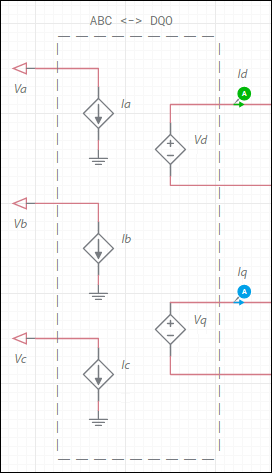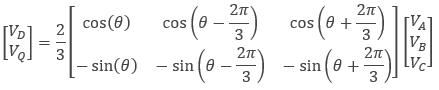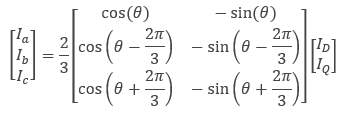OK

# DQ0 coordinate transforms

The DQ0 transform is a space vector transformation of three-phase time-domain signals from a stationary phase coordinate system (ABC) to a rotating coordinate system (DQ0).

Every machine that is modeled in the DQ0 coordinate frame is interfaced to the ABC coordinate frame (the real world) using the following ABCDQ0 transform structure:The matrix representing the expressions for the VD and VQ controlled voltage sources is:The matrix representing the expression for the Ia, Ib, and Ic controlled current sources is:If the reference frame is stationary, then θ is always zero. This is the case for the Induction machine squirrel cage model in Multisim.

If the reference frame is rotating synchronously with the rotor, then θ is the instantaneous mechanical angle of the rotor. This is the case for the Synchronous permanent magnet model in Multisim.